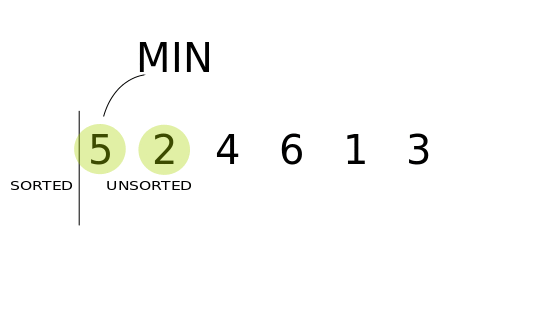# Selection Sort In Python

In a nutshell, sorting is nothing but arranging data in a particular fashion. Selection sort is a popular sorting algorithm.

In Selection sort, First and foremost the list is divided into two parts left part being the sorted which is initially empty and right part unsorted which at the very beginning is the entire list then at each step elements are recursively compared and swapped depending on the condition till the list is entirely sorted.

While sorting a list in ascending order first, we need to go through the entire list and then find the minimum element and swap it with the element in the leftmost. Next, we need to find the second smallest element and swap it with the 2nd element of the list, and this keeps on going until the entire list is sorted.

Similarly, for sorting a list or array in descending order, we need to find the maximum element and swap it with the first element and so on.

Since at each step we are selecting the next element for the sorted list hence named selection sort.Below## Algorithm For Selection Sort In Python

• Step 1Select the minimum element of the list and swap it with the first element (for Ascending order).
• Step 2: In every comparison, if any element is found smaller than the selected element, then both are swapped.
• Step 3: Repeat the same procedure with the element in the next position in the list until the entire list is sorted.

## Python Program For Selection Sort

``````# Sorting function
def selection_sort(l):

for start in range(len(l)):
min_pos = start

for i in range(start, len(l)):
if l[i] < l[min_pos]:
min_pos = i
# swap values
(l[start], l[min_pos]) = (l[min_pos], l[start])

# Test input
l = [54, 26, 93, 17, 77, 31, 44, 55, 20]
selection_sort(l)
print(l)
``````

Output:

``[17, 20, 26, 31, 44, 54, 55, 77, 93]``

## Explanation:

In the above program, the `start` position is initially 0  at each iteration it keeps increasing till the limit `len(l)`, at every iteration when the nested loop finds a smaller element than the element at the position `start` the values get swapped this continues until the entire list is sorted.

## Analysis Of Selection Sort

For an unsorted sequence of length `n` the algorithm requires `n` step for the first scan then at each iteration it reduces by 1. Mathematically this can be expressed as,

``T(n) = n + (n-1) + (n-2) + ....+ 1 = n(n+1)/2 = O(n2)``

The above expression concludes that this algorithm is proportional to n2. Therefore for a given list of size n, the time complexity of selection sort can be expressed as,

Worst Case Time Complexity [ Big-O ]: O(n2)

Best Case Time Complexity [Big-omega]: O(n2)

Average Time Complexity [Big-theta]: O(n2)

Space Complexity: O(1)

PYTHON

# Latest Articles

Latest from djangocentral

## Capturing Query Parameters of request.get in Django

In Django, the request object contains a variety of information about the current HTTP request, including the query parameters. Query parameters are a way to pass additional information in the URL and are used to filter or sort data. The request object p…